Ex 2.2

Chapter 2 Class 12 Inverse Trigonometric Functions (Term 1)
Serial order wise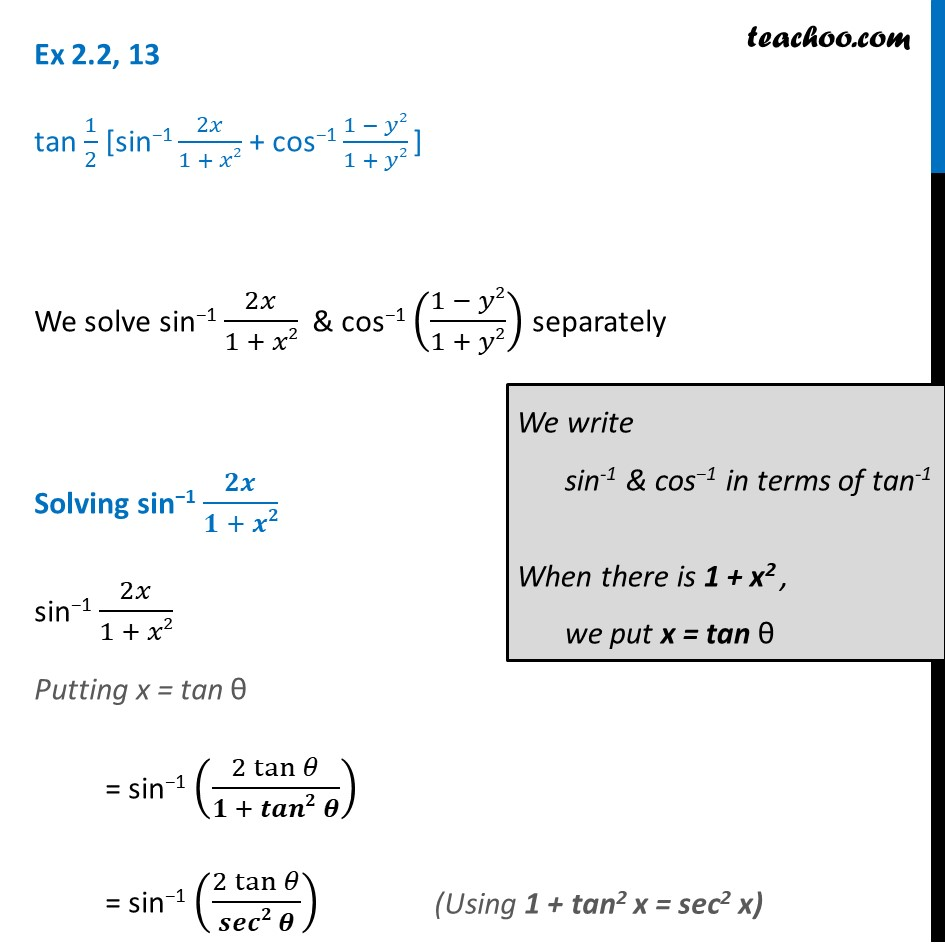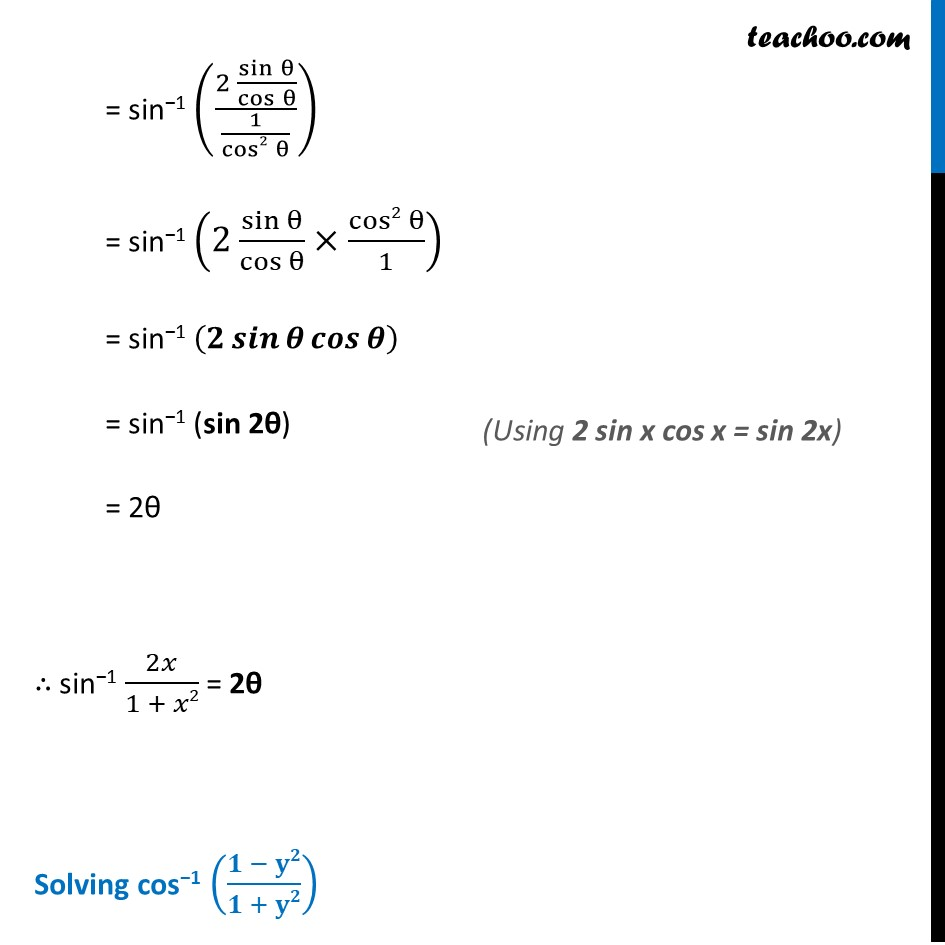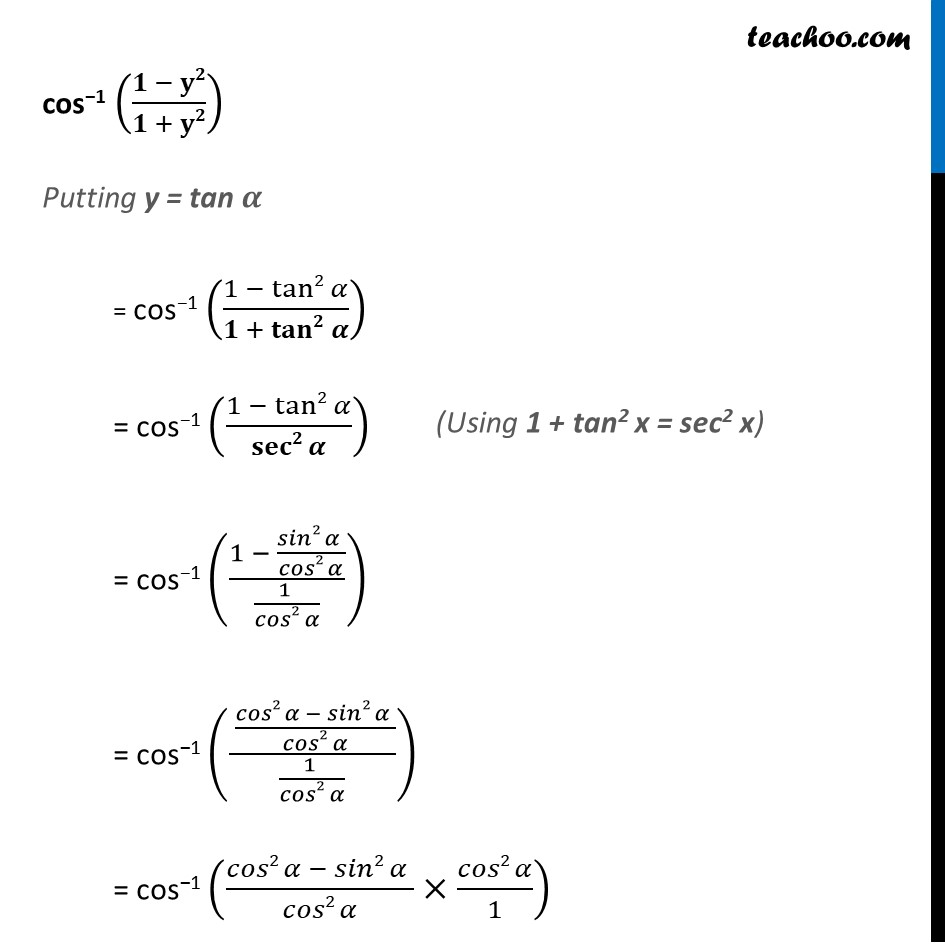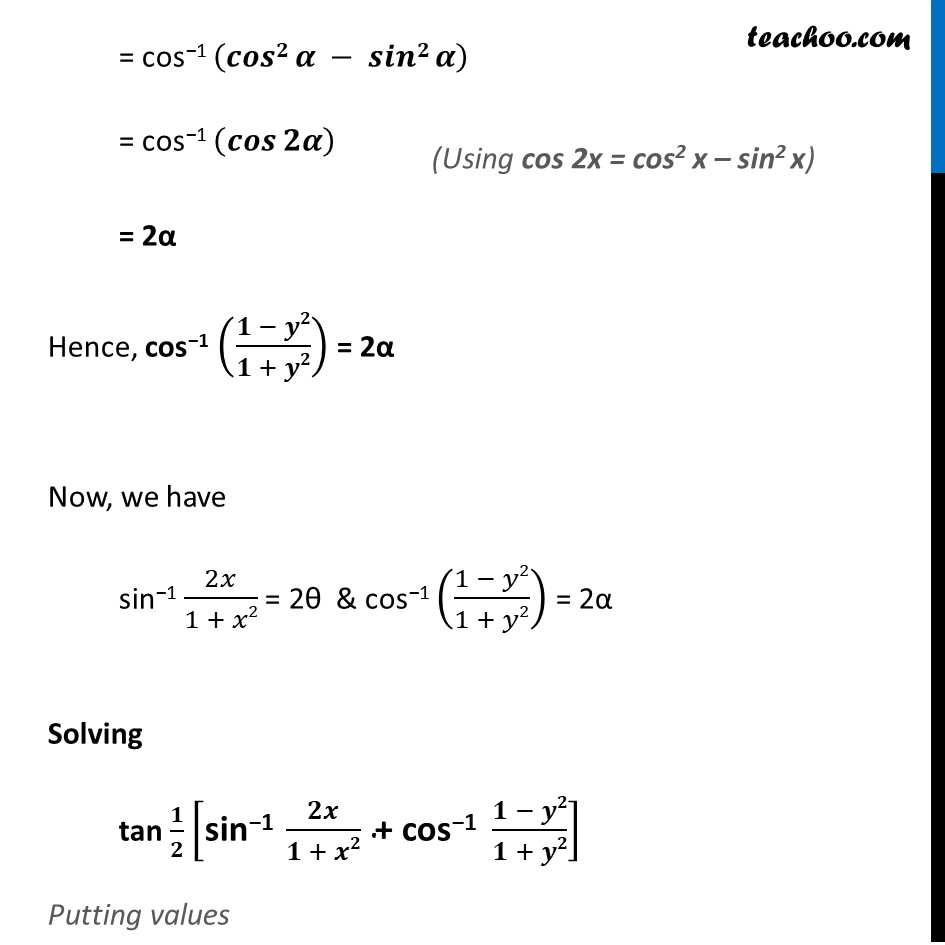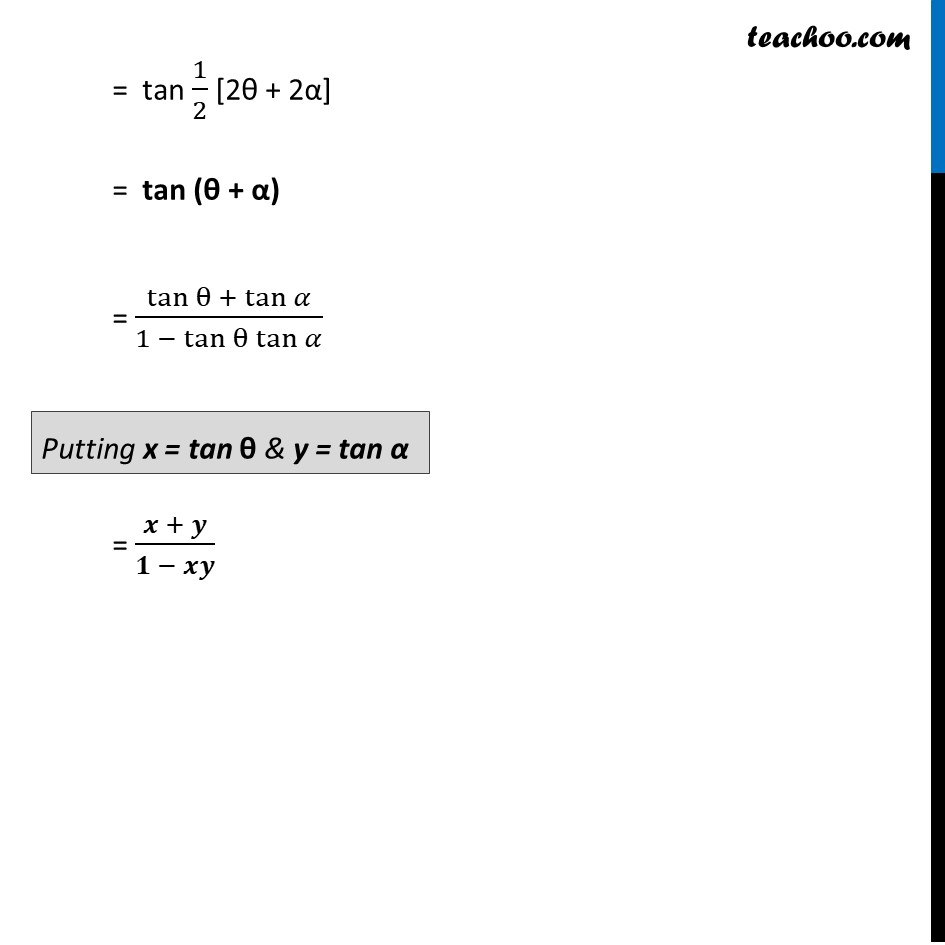### Transcript

Ex 2.2, 13 tan 1/2 [sin−1 2𝑥/(1 + 𝑥2) + cos−1 (1 − 𝑦2)/(1 + 𝑦2) ] We solve sin−1 2𝑥/(1 + 𝑥2) & cos−1 ((1 − 𝑦2)/(1 + 𝑦2)) separately Solving sin−1 𝟐𝒙/(𝟏 + 𝒙𝟐) sin−1 2𝑥/(1 + 𝑥2) Putting x = tan θ = sin−1 ((2 tan⁡𝜃)/(𝟏 + 𝒕𝒂𝒏𝟐 𝜽)) = sin−1 ((2 tan⁡𝜃)/(𝒔𝒆𝒄𝟐 𝜽)) We write sin-1 & cos−1 in terms of tan-1 When there is 1 + x2 , we put x = tan θ (Using 1 + tan2 x = sec2 x) = sin−1 ((2 sin⁡θ/cos⁡θ )/(1/cos2⁡θ )) = sin−1 (2 sin⁡θ/cos⁡θ ×cos2⁡θ/1) = sin−1 (𝟐 𝒔𝒊𝒏⁡𝜽 𝒄𝒐𝒔⁡𝜽 ) = sin−1 (sin 2θ) = 2θ ∴ sin−1 2𝑥/(1 + 𝑥2) = 2θ Solving cos−1 ((𝟏 − 𝐲𝟐)/(𝟏 + 𝐲𝟐)) (Using 2 sin x cos x = sin 2x) cos−1 ((𝟏 − 𝐲𝟐)/(𝟏 + 𝐲𝟐)) Putting y = tan 𝜶 = cos−1 ((1 −〖 tan2〗⁡𝛼)/(𝟏 +〖 𝐭𝐚𝐧𝟐〗⁡𝜶 )) = cos−1 ((1 −〖 tan2〗⁡𝛼)/𝐬𝐞𝐜𝟐⁡𝜶 ) = cos−1 ((1 − (𝑠𝑖𝑛2 𝛼 )/(𝑐𝑜𝑠2 𝛼))/((1 )/(𝑐𝑜𝑠2 𝛼))) = cos−1 (( (𝑐𝑜𝑠2 𝛼 − 𝑠𝑖𝑛2 𝛼 )/(𝑐𝑜𝑠2 𝛼))/((1 )/(𝑐𝑜𝑠2 𝛼))) = cos−1 ((𝑐𝑜𝑠2 𝛼 − 𝑠𝑖𝑛2 𝛼 )/(𝑐𝑜𝑠2 𝛼)×(𝑐𝑜𝑠2 𝛼)/1) = cos−1 (𝒄𝒐𝒔𝟐 𝜶 − 𝒔𝒊𝒏𝟐 𝜶) = cos−1 (𝒄𝒐𝒔⁡𝟐𝜶 ) = 2α Hence, cos−1 ((𝟏 − 𝒚𝟐)/(𝟏 + 𝒚𝟐)) = 2α Now, we have sin−1 2𝑥/(1 + 𝑥2) = 2θ & cos−1 ((1 − 𝑦2)/(1 + 𝑦2)) = 2α Solving tan 𝟏/𝟐 ["sin−1 " 𝟐𝒙/(𝟏 + 𝒙𝟐) " + cos−1 " (𝟏 − 𝒚𝟐)/(𝟏 + 𝒚𝟐)] Putting values (Using cos 2x = cos2 x – sin2 x) = tan 1/2 [2θ + 2α] = tan (θ + α) = (tan⁡θ + tan⁡𝛼)/(〖1 − tan〗⁡θ tan⁡𝛼 ) = (𝒙 + 𝒚)/(𝟏 − 𝒙𝒚) Putting x = tan θ & y = tan α